# Calculating the Rate an Investment Achieves Using the RRI Function

We have already seen how to use the PV Function, the FV Function and the PDURATION Function in a previous tutorial. We have also seen how to calculate NPV and XNPV and the difference between the two, in another tutorial.

We are now, going to look at how to calculate the equivalent interest rate for the growth of an investment. Put more simply, if we are given a present value, a future value and the number of periods for an investment, we can calculate the rate of return for those periods using the RRI function. The RRI Function was introduced in Excel 2013 and allows one to calculate this compounding interest rate for an investment.

Let’s remind you of a few financial concepts needed for this calculation.

PV or present value is the value of the investment or loan at the beginning of its lifespan.

FV or future value is the value of the investment or loan at some time in the future.

The number of periods or nper is the total number of periods (each period is a payment) between the present value and future value of a loan/investment. Payments are usually monthly, especially in the case of loans. Although, years can be input directly, into this particular function as well.

The syntax for the RRI Function is: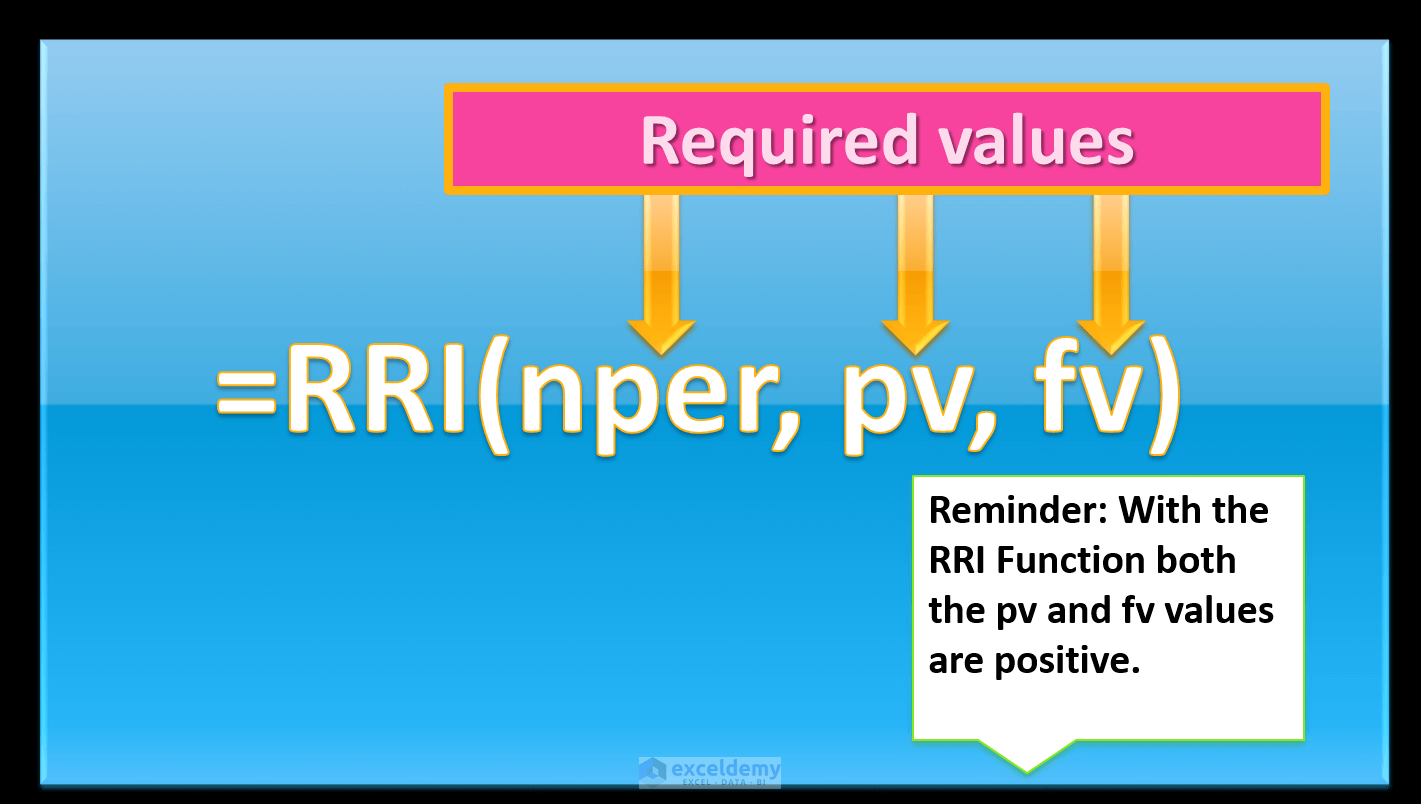## Calculating RRI

The present value of an investment is \$1000. The future value is \$3000, and the number of monthly periods is 48 months (i.e over a 4-year duration).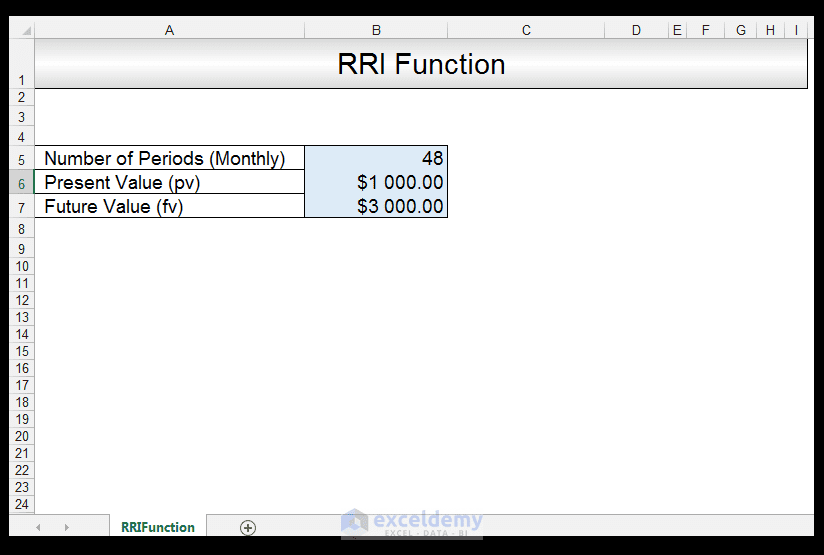1) In order to calculate the RRI, in Cell B8 we input the following formula:

=RRI (B5, B6, B7)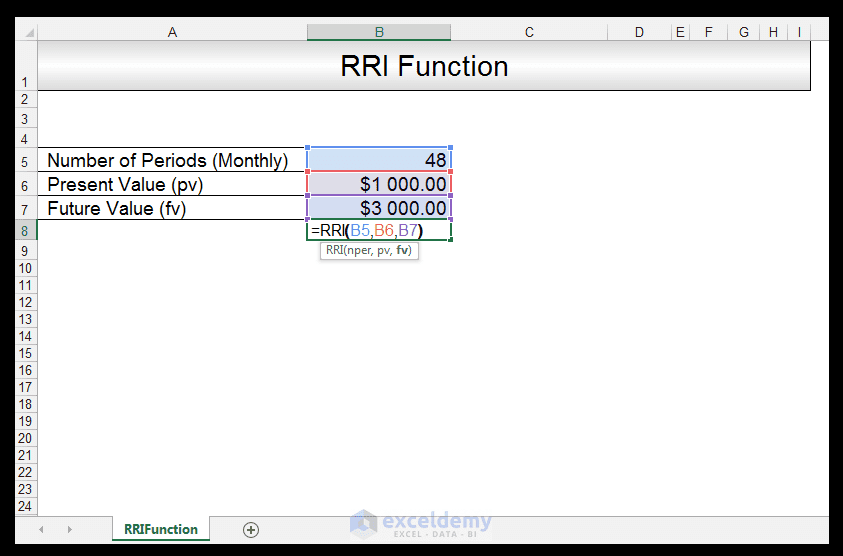2) Upon pressing CTRL-ENTER, we get the value of 0.02315169.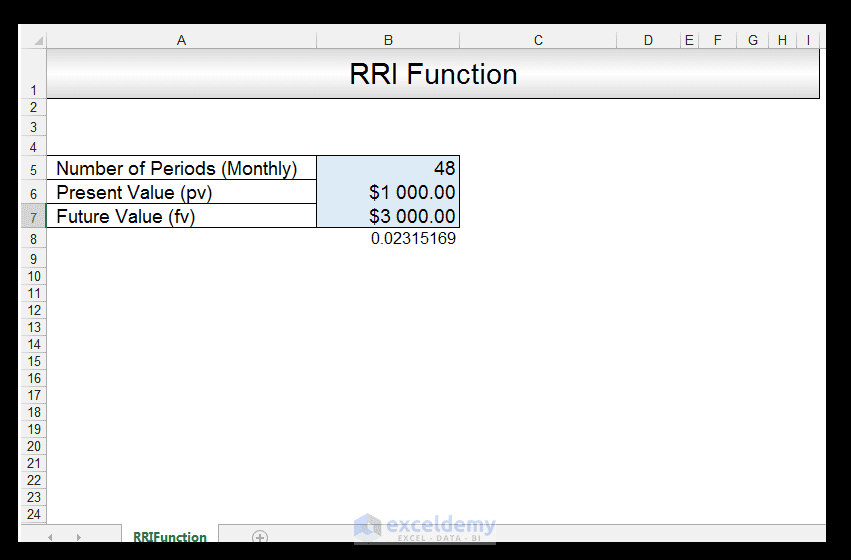3) In order to see this as a percentage, we right-click the cell, choose Format Cells and then format as a percentage with two decimal places. Click Ok.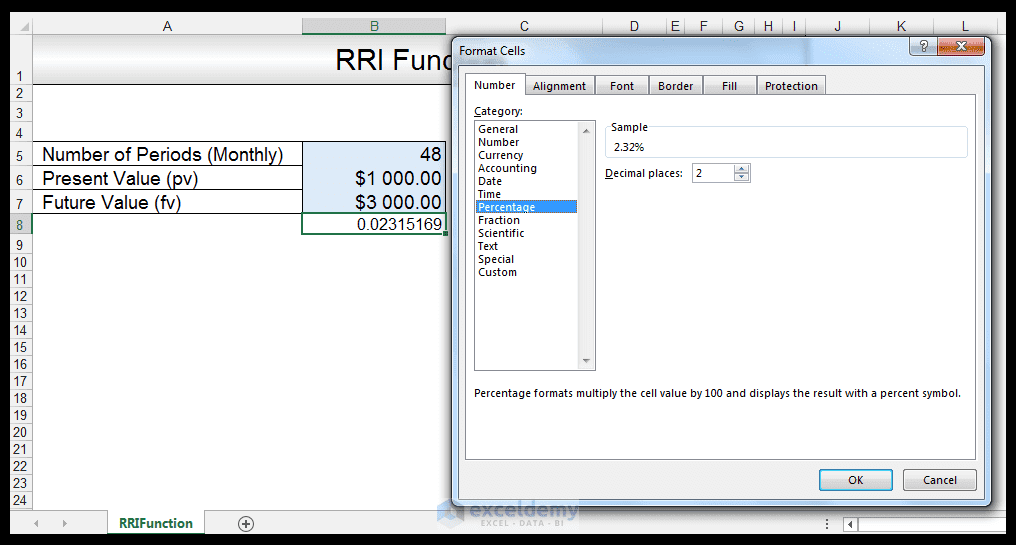4) The equivalent interest rate is now shown as a percentage.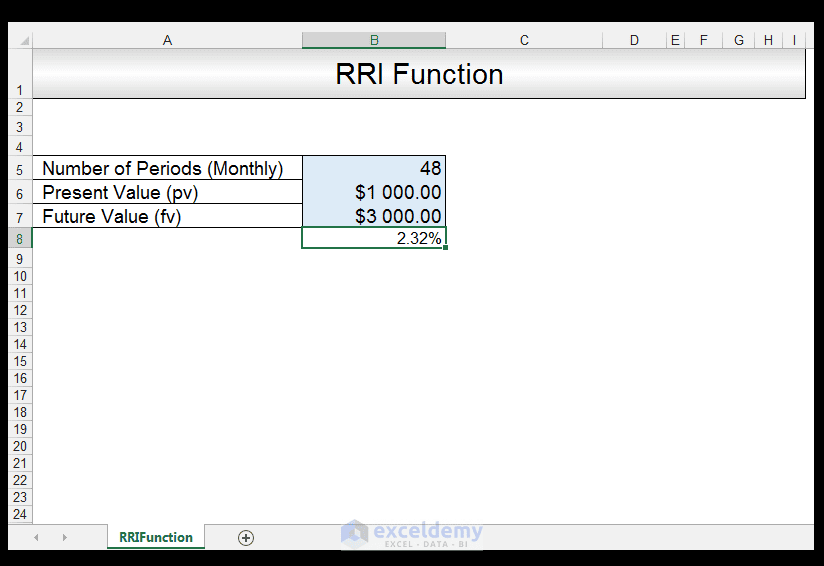And there you have it. The equivalent rate for the growth of an investment that started out with a present value of \$1000, with a future value of \$3000, whose monthly periods was 48 months is 2.32 %.

## Conclusion

The RRI Function is a useful function that was introduced in 2013, that allows one to calculate the equivalent rate of an investment. An interesting facet of this function is that both present value and future value are input as positives, which is unlike the logic needed for some other financial functions in Excel.

It is also important to note that the RRI Function, is used for relatively simple interest rate calculations. For example, where one already knows the present value of an investment from the start and its future value and of course its entire duration. For more complex situations, involving depreciation or appreciation, or when one is handling additional expenses or earnings during the course of an investment, more complex financial functions and calculations are needed.

Please feel free to comment and tell us if you use the RRI function in your financial spreadsheets.

The PV, FV and PDURATION Excel Functions Tutorial

Optimizing an investment portfolio using Excel Solver

NPV versus XNPV Excel Functions Tutorial

How to Calculate Variance in Excel

Compound interest excel formula with regular deposits